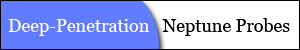"Penetrating Planets Since 2004"ECE6390 Introduction to Satellite Communications Fall, 2004
Initial ResearchIntroductionProject RequirementsNeptune FactsCalculations
Penetrating ProbesIntroductionDesignCalculationsParts & Pricing
Relay SatelliteIntroductionLink with ProbeCalculationsLink with EarthCalculationsParts & Pricing
ConclusionsSummaryTotal CostContact Info
Home » Penetrating Probes » Calculations
 Calculations   Following the block diagram, the signal is digital until the BPSK modulator where it is then converted into analog.  Most of these digital components have some sort of regenerative devices to help sharpen the digital signals, eliminating any effective noise in the system.  Once in the analog format, it is also acceptable to eliminate noise from the calculations because the signal is being powered by a fairly high power source (Watts compared to femtoWatts) and will not affect the carrier signal. As mentioned before, the power amplifier is capable of transmitting a constant 20W output, which results in a 20W signal being transmitted through the dish antenna.  The dish antenna used in this project was rated at 28 dBi gain at 2.4 GHz.  Usingthe efficiency of the satellite dish was calculated to be 85%.  Then, using this equation again with a frequency of 500 MHz, the gain of the antenna was determined to be 15.2638 dBi. Using the newly-determined gain of the dish antenna, the power leaving the probe could be calculated byIn the worst case, this signal will come from an off-angle probe at a distance of 420km into the atmosphere.  As seen in the attenuation data of the (see Penetrating Probes » Introduction), the attenuation 420km plus the extra distance due to a 45° angle to the relay satellite is calculated to be 4.72 dB.  From a quick observation, it is evident that most of the loss will occur from the propogational loss to the relay satellite once the signal has emerged from the atmosphere.  To continue in the link budget, the power received at the relay satellite is determined byWhere λ is the wavelength at 500 MHz and R is the free-space distance from the probe to the satellite (67,565km).  While this power seems quite small, it is important to know that with spread spectrum, it is possible for the spreaded signal to lay below the noise floor and still be able to be received. For the continuation of this link, please go to the Relay Satellite » Introduction section to see how the signal is received and decoded.     Next Section » References:
top of page

William W
e-mail